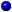SYLLABUS  Previous: 9.2 Programming in JAVA  Up: 9 PROBLEM BASED LEARNING  Next: 9.4 Interactive evaluation form

## 9.3 Parameters and switches in HTML

Slide : [ - || JBONE tree - names || JAVA tutorial ]Selectors.

The selectors appear on the top of JBONE plot window and allow you to define the type of problem you want to solve. Careful, white spaces here do count!
• method selects the numerical method. Choices include
"Finite differences", "Finite elements", "Fourier transform", "Lagrangian methods", "Particle methods","Particle methods*", "Sampling methods"."Sampling methods*".
• scheme selects a particular scheme in a given method. Choices include
"Standard scheme", "Explicit 2-level", "Explicit 3-level", "Implicit 2-level", "Expl-LaxWendroff", "Impl-LaxWendroff", "Lax-Friedrichs", "Leap-frog (FDTD)", "European naive", "European vanilla", "American naive", "American vanilla", "Tune Integration", "Expanded convol.", "Aliased convol.", "CubicHermite FEM", "Cubic--Splines", "MC norm. walk", "MC log-normal", "My scheme", "Exercise 2.01", "Exercise 2.03", etc
• ic selects the type of initial condition. Choices include
"Box", "Gaussian", "Cosine", "Soliton", "WavePacket", "PutOption".
• pde selects the type of PDE. Choices include
"Advection", "Burger (shock)", "KdV (solitons)", "Schroedinger", "Black-Scholes", "My equation 1", "My equation 2", "Exercise".Editable parameters.

The following list of input parameters can be used to change the defaults pre-defined in the JBONE code:
• Velocity the advection velocity (or the market interest rate)
• Diffusion the diffusion (or the market volatility)
• Dispersion the dispersion
• TimeStep the time step
• MeshPoints the number of mesh points
• Walkers the number of particles
• TimeImplicit the time implicit parameter
• TuneIntegr the tunable integration parameter
• ICAmplitude the initial condition amplitude
• ICPosition the initial condition position
• ICWidth the initial condition width
• ICWavelength the initial condition wavelength
• RunTime the physical run time
• MeshLeft the left position of the mesh
• MeshLength the length of the simulation box
• PhysDataCase the type of Physical Data, e.g potential shape
• PhysDataValue a Physical Data parameter, e.g potential amplitudeTAG defaults.

The JBONE applet is included an HTML document with a header of the form
```<applet codebase="\$user_dir/applet/" code=\$applet
align=center width=780 height=400>
<param name=Velocity            value=0.>
<param name=Diffusion           value=0.>
<param name=Dispersion          value=0.>
<param name=TimeStep            value=0.5>
<param name=MeshPoints          value=64>
<param name=Walkers             value=0>
<param name=TimeImplicit        value=0.7>
<param name=TuneIntegr          value=0.333>
<param name=ICAmplitude         value=1.>
<param name=ICPosition          value=18.>
<param name=ICWidth             value=8.>
<param name=ICWavelength        value=4.>
<param name=RunTime             value=128.>
<param name=MeshLeft            value=0.>
<param name=MeshLength          value=64.>
<param name=PhysDataCase        value=1>
<param name=PhysDataValue       value=1.>
<param name=method              value="Finite differences">
<param name=scheme              value="Exercise 2.01">
<param name=ic                  value="Gaussian">
<param name=pde                 value="Exercise">
</applet>
```
where the first two lines specify the path name of the executable, the position and the size of the window. The TAGS that follow define the default values of parameters and switches that are set when the applet appears in the web page.

SYLLABUS  Previous: 9.2 Programming in JAVA  Up: 9 PROBLEM BASED LEARNING  Next: 9.4 Interactive evaluation form